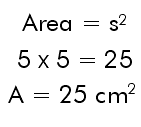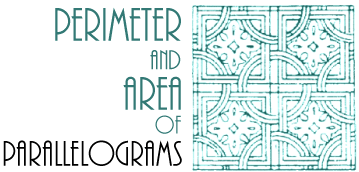By definition, a parallelogram is a quadrilateral with opposite sides parallel and equal.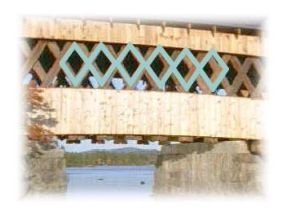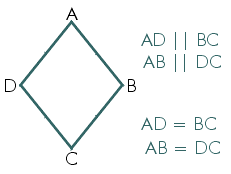To find the perimeter of a parallelogram, one can either add the lengths of all the sides or use these formulas. P = 2L + 2W or P = 2 (L + W)2 x 12 + 2 x 8 =  2(12 + 8) = 40 P = 40 cm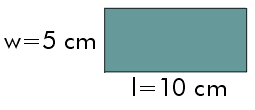To find the area of a rectangle, multiply the length timesthe width.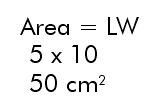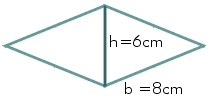To find the area of a parallelogram multiply its base times its height.To find the perimeter of a square multiply the length
of one side by four.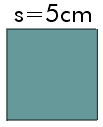4 x 5 = 20cm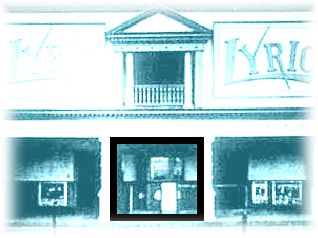To find the area of a square multiply the length of one side by itself.